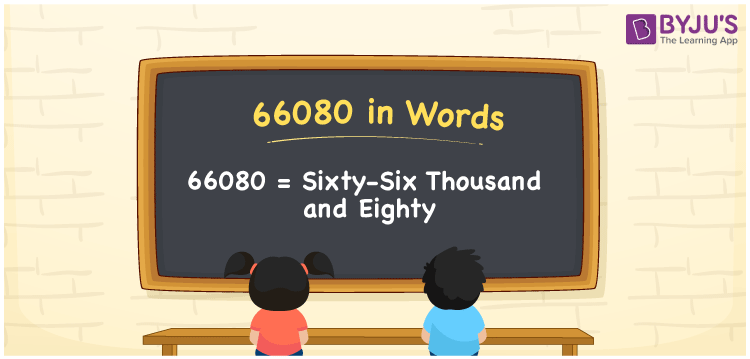# 66080 in Words

66080 in words is written as Sixty-six thousand eighty. In both the International System of Numerals and the Indian System of Numerals, 66080 is written as Sixty-six thousand eighty. The number 66080 is a Cardinal Number as it represents some quantity. For example, “the Smart-TV costs 66080 rupees”, 66080 represents the cost of the TV.

 66080 in Words Sixty-six thousand eighty Sixty-six thousand eighty in Number 66080

## 66080 in English Words

66080 in English words is read as “Sixty-six thousand eighty”## How to Write 66080 in Words?

To write 66080 in words, we shall use the place value chart. In the place value chart, put 6 in the ten thousands, 6 in the thousands, 0 in the hundreds, 8 in the tens and 0 in the ones, respectively. Let us make a place value chart to write the number 66080 in words.

 Ten Thousands Thousands Hundreds Tens Ones 6 6 0 8 0

Thus, we can write the expanded form as

6 × Ten Thousand + 6 × Thousand + 0 × Hundred + 8 × Ten + 0 × One

= 6 × 10000 + 6 × 1000 + 0 × 100 + 8 × 10 + 0 × 1

= 60000 + 6000 + 0 + 80 + 0

= 66080

= Sixty-six thousand eighty.

66080 is a natural number, the successor of 66079 and the predecessor of 66081.

66080 in words – Sixty-six thousand eighty

• Is 66080 an odd number? – No
• Is 66080 an even number? – Yes
• Is 66080 a perfect square number? – No
• Is 66080 a perfect cube number? – No
• Is 66080 a prime number? – No
• Is 66080 a composite number? – Yes

## Frequently Asked Questions on 66080 in Words

Q1

### How to write 66080 in words?

66080 in words is written as Sixty-six thousand eighty.
Q2

### How to write 66080 in the International and Indian System of Numerals?

In both, the system of numerals, 66080 in words, is written as Sixty-six thousand eighty.
Q3

### How to write 66080 in a place value chart?

In the place value chart, write 6 in the ten thousands, 6 in the thousands, 0 in the hundreds, 8 in the tens and 0 in the ones, respectively.